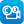# Search results

## Refine by topic

Main topic Specific topic
Related topic
Listed under:  Science  >  Forces and energy  >  Mechanical energy  >  Velocity### Gravity and orbits

This is an interactive teaching and learning resource that years 7 to 10 secondary students can use to simulate the orbits of the Earth, Moon and a space station while altering the physical quantities involved. Orbital pathways, velocity and force vectors can be displayed in either scale or cartoon views. The mass and velocity ...### Laptop wrap: Project projectiles

A laptop-friendly resource focussed on the motion of projectiles, with links to video experiments and interactive websites that can assist students to develop a conceptual understanding of this type of motion.### Measuring Speed

Students use this resource consisting of eleven slides with diagrams, written explanation and voice-over to understand how to work out the speed of a moving object and understand how to make calculations using the relationship between speed, distance and time. There is a two-question quiz and a summary slide.### Distance-time Graphs

Students use this resource consisting of ten slides with diagrams, written explanation and voice-over to understand how to plot a distance-time graph and understand what it shows. There is a two-question quiz and a summary slide.### Measuring Speed (Simulation)

Students use this resource consisting of a webpage with diagrams of three cars that students can vary the speed at which they are travelling. They can calculate the time to travel 3000m and then observe the cars as they travel the distance. This demonstrates how to work out the speed of a moving object and how to make calculations ...